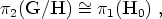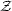### 2. DEFECTS IN THE UNIVERSE

Generically topological defects will be produced if the conditions for their existence are met. Then for example if the unbroken group H contains a disconnected part, like an explicit U(1) factor (something that is quite common in many phase transition schemes discussed in the literature), monopoles will be left as relics of the transition. This is due to the fundamental theorem on the second homotopy group of coset spaces [Mermin, 1979], which states that for a simply-connected covering group G we have (5)(4)

with H0 being the component of the unbroken group connected to the identity. Then we see that since monopoles are associated with unshrinkable surfaces in G/H, the previous equation implies their existence if H is multiply-connected. The reader may guess what the consequences are for GUT phase transitions: in grand unified theories a semi-simple gauge group G is broken in several stages down to H = SU(3) × U(1). Since in this case1(H), the integers, we have2 (G / H)1 and therefore gauge monopole solutions exist [Preskill, 1979].

5 The isomorfism between two groups is noted as. Note that by using the theorem we therefore can reduce the computation of2 for a coset space to the computation of1 for a group. A word of warning: the focus here is on the physics and the mathematically-oriented reader should bear this in mind, especially when we will become a bit sloppy with the notation. In case this happens, consult the book [Steenrod, 1951] for a clear exposition of these matters. Back.